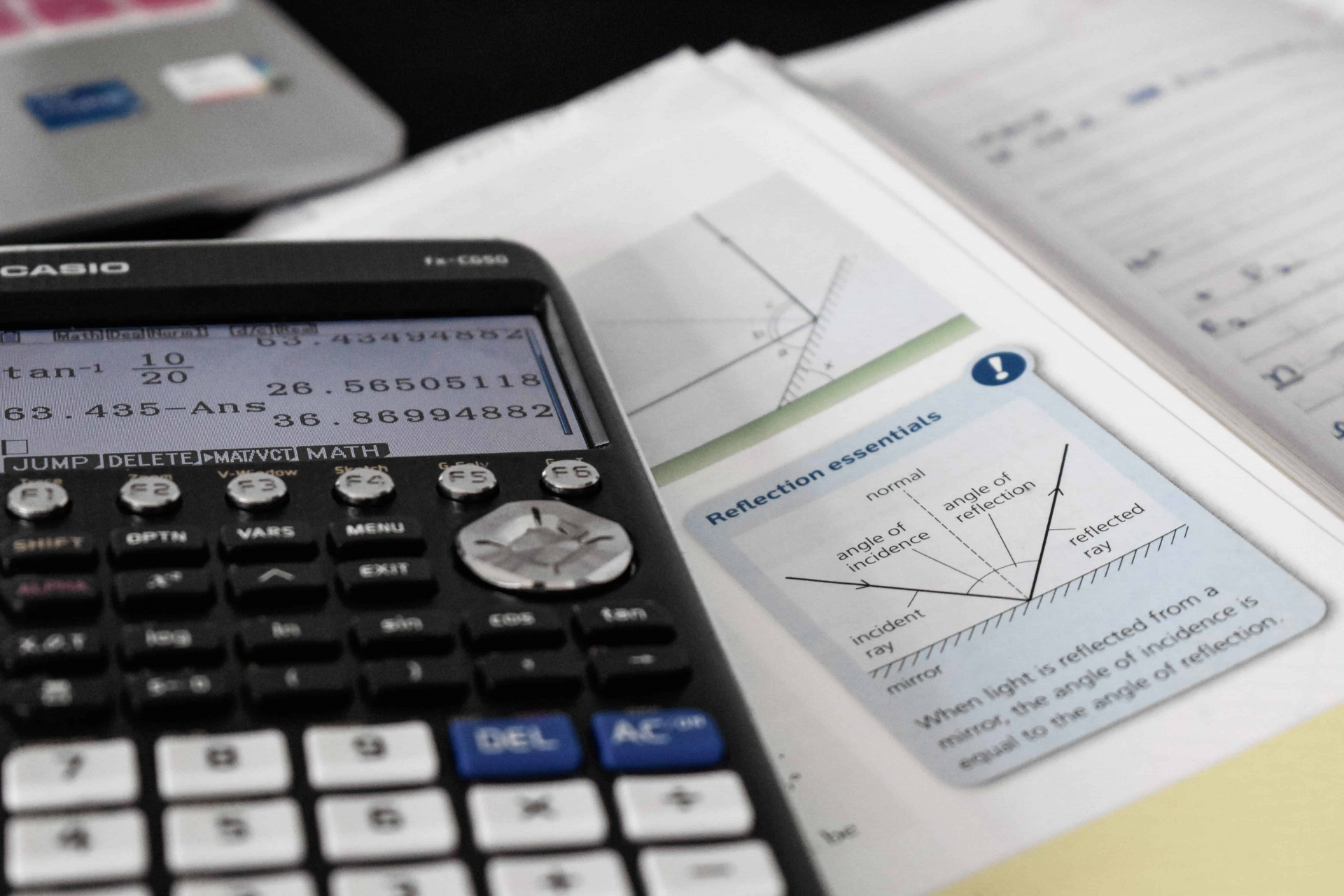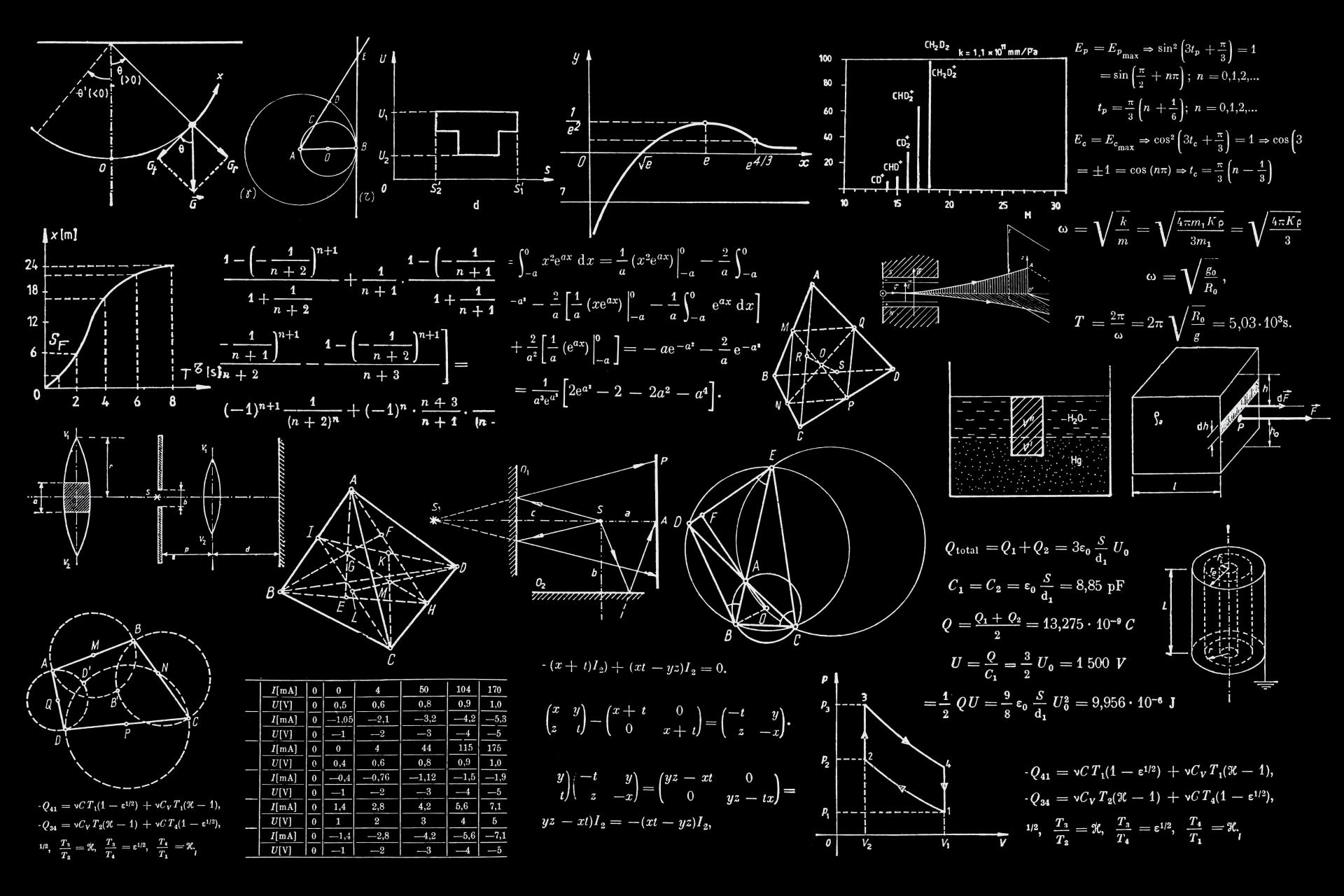To understand abstract algebra you first need to understand what algebra is. Algebra is a branch of mathematics that studies mathematical symbols and the rules to manipulate these symbols in formulas.

Within the algebra umbrella, there are more specific fields. For example, there is elementary algebra that dedicates to manipulating variables as if they were numbers and is therefore essential in all applications of mathematics.

There's also linear algebra that deals with linear equations and linear mappings. It is used for modern presentations of geometry and has many practical applications (in weather forecasting, for example). Finally, there's abstract or modern algebra, which studies algebraic structures such as groups, rings, and fields.

Keep in mind that the word algebra is not only used to refer to an area in math. It can also be used to name some sorts of algebraic structures, such as an algebra over a field, commonly called an algebra.

Sometimes, the same phrase is used for a subarea and its main algebraic structures; for example, Boolean algebra and a Boolean algebra. A mathematician specializing in algebra is called an algebraist.The best Mathematics tutors available5 (71 reviews)
Davayne
\$20
/h1st lesson free!5 (23 reviews)
Lyle
\$36
/h1st lesson free!5 (39 reviews)
Cassandre
\$30
/h1st lesson free!5 (27 reviews)
Jeffry
\$88
/h1st lesson free!5 (18 reviews)
Anh
\$30
/h1st lesson free!5 (17 reviews)
Reza
\$33
/h1st lesson free!5 (8 reviews)
Bryan
\$35
/h1st lesson free!5 (20 reviews)
Momin khan
\$20
/h1st lesson free!5 (71 reviews)
Davayne
\$20
/h1st lesson free!5 (23 reviews)
Lyle
\$36
/h1st lesson free!5 (39 reviews)
Cassandre
\$30
/h1st lesson free!5 (27 reviews)
Jeffry
\$88
/h1st lesson free!5 (18 reviews)
Anh
\$30
/h1st lesson free!5 (17 reviews)
Reza
\$33
/h1st lesson free!5 (8 reviews)
Bryan
\$35
/h1st lesson free!5 (20 reviews)
Momin khan
\$20
/h1st lesson free!

## What is the history of abstract algebra?

Algebra has been around since the beginning of time, as well as mathematics. However, it hasn't always been called that, and its roots have shaped the field into what we know today.

Algebra comes from an Arabic word that means 'reunion of broken parts.' The term and the field of study originated from an ancient Arabic book called 'The Science of Restoring and Balancing' by Persian mathematician and astronomer al-Khwarizmi.

In his work, al-Khwarizmi used the term to refer to the operation of solving things by moving a term from one side of an equation to the other, and "balancing" inequalities.You can learn about exponents, lines in a graph (slope), or solving an equation with the help of an app. Photo by Anoushka P on Unsplash

The roots of algebra can also be traced to the ancient Babylonians, who worked on creating an arithmetical system to do calculations algorithmically.

The Greeks invented geometric algebra where terms were represented by sides of geometric objects, usually lines, that had letters associated with them.

From the development of algebra, linear equations, and expressions with different variables, there was a shift in this methodology of mathematics during the end of the 19th century and the early 20th century.

During this period of time, the field of abstract or modern algebra was born. Mathematicians were unsatisfied with establishing the properties of concrete objects, which is why they turned their attention to general theory. In the 19th century, formal definitions of several algebraic structures started to appear.

The work of mathematicians like Ernst Steinitz, David Hilbert, Emil Artin, Emmy Noether, Leopold Kronecker, Richard Dedekind, and many more came to define abstract algebra. Each mathematician worked on different notions but each branch together became abstract algebra.

If you aren't already studying with the help of a tutor, we recommend you search for tutors on Superperof. A tutor can help you solve your homework, and teach you about fractions, polynomial equations, functions, quadratic expressions, and more.

Some tutors offer classes online or in person. It depends on the modality you like best for your math class. Online lessons are more accessible because no one has to commute anywhere. However, an in-person class allows for better communication and an easier exchange of information.

Learning algebra or math is about learning how to solve different problems. Each time math becomes more complicated because more variables are added to each problem, but the bottom line is you always have to solve problems.

## What are the elements of abstract algebra?

The subject has a wide variety of elements, however, the three principal elements are group theory, Galois theory, and classical ideal theory. Each of these topics covers several important subtopics that makeup what we know as abstract or modern algebra.

The elements of abstract algebra are variable and real, and to understand them we recommend you ask your teachers for a book you could use to study and solve problems.

There are a number of options in the market, but algebra teachers usually recommend Elements of Abstract Algebra written by Allan Clarke. The author focuses on number theory and solvability by radicals. Each chapter of the book covers different mathematical topics stemming from set theory, group theory, ring theory, and field theory.

It emphasizes the definition of normal subgroups, permutation groups, and composition series in group theory, polynomial rings and ideals in ring theory, and algebraic numbers and Galois theory of field theory.

## What is the difference between abstract and linear algebra?

Students usually get confused understanding the different branches and sub-subjects of math because they aren't clear on what exactly each branch is dedicated to.

As you could see, abstract algebra studies algebraic structures such as groups, rings, and fields.

On the other side, linear algebra concerns linear equations, linear maps, and their representations in vector spaces and matrices. Vectors are understood as quantities having both magnitude and direction and are often expressed using coordinates.

For example, consider a solid object that is free to move in any direction. When two forces act at the same time on this object, they produce a combined effect that is the same as a single force.

Both fields are dedicated to solving algebraic inequalities but that doesn't mean that they study the same thing. Each field studies different areas. Abstract algebra studies groups, rings, and fields, whereas linear algebra focuses on lines.

Linear algebra is central to many other branches of mathematics. For example, it is fundamental in modern presentations of geometry. It is also used in other fields like engineering because it allows modeling many natural phenomena, and computing efficiently with such models.

Students typically hire online tutors to help them learn the subject much faster. Keep in mind that teachers and tutors are usually experts in their field and they are capable to teach solving exponents, fractions, roots, equations, graphs, problems, quadratic variables, polynomial equations, and every branch of algebra.

This means you can find one person to teach you everything you need to know about math. You can study with the same tutor for years and review your progress at the end of the school year.

## Is abstract algebra hard to learn?During a math lesson, you will find the solution to rational problems, graphs and the correct slope, and more. Photo by Dan Cristian Pădureț on Unsplash

Learning anything new takes time, it is not like you can learn about functions, expressions, mathematical inequalities, roots, fractions, exponents, geometry, problems, and more in one day.

Learning is about doing things one step at a time. You can take things day by day and focus on whatever your teachers are telling you to focus on.

Students tend to want things fast and easy because technology today does everything fast and easy. This is why, when faced with a problem or a challenge, they tend to feel like a failure when they don't fix it after a couple of hours of trying.

Students can now download a free app and learn things in school fast and easily. This is why so many struggles and quit when faced with a problem they can't solve fast.

However, learning math is challenging and there is no way around it. You either learn how to do solve the quadratic equation, what each variable stands for, how to interpret a graph, and what is a rational number, o a polynomial, or you won't do well in class.

If you want to learn abstract algebra and make it easy, we recommend you consider hiring a private tutor who can help you outside school hours.

During each lesson, you can review your class notes, ask questions, solve different mathematical expressions, functions, and inequalities with help, and find a form to make each lesson fun.

A tutor will take their student step by step and provide guidance and support through their learning process. Students that struggle with math class can benefit from having a tutor because they'll be able to dominate their fears and pass the class with ease.

Don't forget that you can search for a tutor on Superprof and see if they offer their first lesson for free. During that first free lesson, you can pay attention to their teaching methods, ask all the questions you might have, and more.

Make sure you are confident to work and study with the tutor you pick!The platform that connects tutors and students5.00 (1 rating(s))Loading...